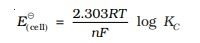# Derivation of Nernst Equation

According to the Nernst Equation, for electrode reaction $Mn^{+}(aq)\; + \;ne \rightarrow \; M(s)$, the electrode potential at any specific concentration measured w.r.t standard hydrogen electrode (SHE) can be represented as:Since, the concentration of solids is taken as unity, therefore, M = 1Where,

$E^{\theta}_{(M^{n+}/M)}$ =  Electrode potential at any concentration measured w.r.t SHE

R = Gas constant = 8.314 $JK^{-1} mol^{-1}$,

F = Faraday constant = 96487 $C \;mol^{-1}$,

T = Temperature (Kelvin), and

[Mn+] = Concentration of species

For a general electrochemical reaction of the type:

$aA + bB \overset{ne}{\rightarrow} cC + dD$

The Nernst equation can be represented as:Equilibrium Constant from Nernst Equation:Thus, the above equation shows a relationship between the standard potential of the cell and equilibrium constant of the reaction in which that reaction takes place. Thus, the equilibrium constants of any reaction, can be measured from the corresponding E° value of the cell.

Applications of Derivation of Nernst Equation:

1. Applications of Derivation of Nernst Equation:
2. 1.One of the major application of Nernst equation is in determining ion concentration
3. 2.It is used to estimate the potential of an ion of charge “z” across a membrane.
4. 3.It is used in oxygen and the aquatic environment.
5. 4.It is also used in solubility products and potentiometric titrations.
6. Nernst Equation is also used in pH measurements.

#### Practise This Question

In which of the following life stages of the silk moth is silk extracted?